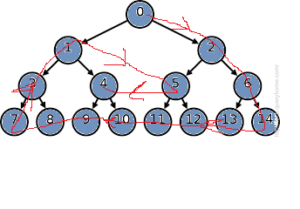# Clockwise Triangular traversal of a Binary Tree

Given a Complete Binary Tree, the task is to print the elements in the Clockwise traversal order.

Clockwise Traversal of a tree is defined as:For the above binary tree, the Clockwise Traingular traversal will be
0, 2, 6, 14, 13, 12, 11, 10, 9, 8, 7, 3, 1, 5, 4

Examples:

```Input:
1
/       \
2         3
/   \      /   \
4     5    6    7
/ \    /\
8   9  10 11
Output: 1, 3, 7, 11, 10, 9, 8, 4, 2, 6, 5

Input:
1
/   \
2     3
Output: 1, 3, 2
```

## Recommended: Please try your approach on {IDE} first, before moving on to the solution.

Approach:

Create a vector tree[] where tree[i] will store all the nodes of the tree at level i. Take an integer k which keeps track which level we are traversing other integer and cycle in which keep tracks how many cycles have been completed. Now, start printing the nodes the rightmost remaining node which has not been traversed yet & keep moving down until you reach down to the last level which has not been traversed now print this level from right to left, then move print leftmost remaining leftmost element of each level starting from last level to moving to the uppermost level whose elements has all not been traversed yet, now again do the same thing until all elements have not been traversed.

Below is the implementation of the above approach:

 `// C++ program for the ` `// above approach ` `#include ` `using` `namespace` `std; ` ` `  `// Function to create an ` `// edge between two vertices ` `void` `addEdge(``int` `a, ``int` `b, vector<``int``> tree[]) ` `{ ` ` `  `    ``// Add a to b's list ` `    ``tree[a].push_back(b); ` ` `  `    ``// Add b to a's list ` `    ``tree[b].push_back(a); ` `} ` ` `  `// Function to create ` `// complete binary tree ` `void` `createTree(``int` `n, vector<``int``> tree[]) ` `{ ` `    ``for` `(``int` `i = 1;; i++) { ` `        ``// Adding edge to ` `        ``// a binary tree ` `        ``int` `c = 0; ` `        ``if` `(2 * i <= n) { ` `            ``addEdge(i, 2 * i, tree); ` `            ``c++; ` `        ``} ` `        ``if` `(2 * i + 1 <= n) { ` `            ``addEdge(i, 2 * i + 1, tree); ` `            ``c++; ` `        ``} ` ` `  `        ``if` `(c == 0) ` `            ``break``; ` `    ``} ` `} ` ` `  `// Modified Breadth-First Function ` `void` `bfs(``int` `node, vector<``int``> tree[], ``bool` `vis[], ` `         ``int` `level[], vector<``int``> nodes[], ``int``& maxLevel) ` `{ ` ` `  `    ``// Create a queue of ` `    ``// {child, parent} ` `    ``queue > qu; ` ` `  `    ``// Push root node in the front of ` `    ``// the queue and mark as visited ` `    ``qu.push({ node, 0 }); ` `    ``nodes.push_back(node); ` `    ``vis[node] = ``true``; ` `    ``level = 0; ` ` `  `    ``while` `(!qu.empty()) { ` ` `  `        ``pair<``int``, ``int``> p = qu.front(); ` ` `  `        ``// Dequeue a vertex ` `        ``// from queue ` `        ``qu.pop(); ` `        ``vis[p.first] = ``true``; ` ` `  `        ``// Get all adjacent vertices of the dequeued ` `        ``// vertex s. If any adjacent has not ` `        ``// been visited then enqueue it ` `        ``for` `(``int` `child : tree[p.first]) { ` `            ``if` `(!vis[child]) { ` `                ``qu.push({ child, p.first }); ` `                ``level[child] = level[p.first] + 1; ` `                ``maxLevel = max(maxLevel, level[child]); ` `                ``nodes[level[child]].push_back(child); ` `            ``} ` `        ``} ` `    ``} ` `} ` ` `  `// Function to display the pattern ` `void` `display(vector<``int``> nodes[], ``int` `maxLevel) ` `{ ` ` `  `    ``// k represents the level no. ` `    ``// cycle represents how many ` `    ``// cycles has been completed ` `    ``int` `k = 0, cycle = 0; ` ` `  `    ``// While there are nodes ` `    ``// left to traverse ` `    ``while` `(cycle - 1 <= maxLevel / 2) { ` ` `  `        ``// Traversing rightmost element ` `        ``// in each cycle as we move down ` `        ``while` `(k < maxLevel - cycle) { ` `            ``int` `j = nodes[k].size() - 1; ` `            ``cout << nodes[k][j - cycle] << ``" "``; ` `            ``k++; ` `        ``} ` ` `  `        ``// Traversing each element of remaing ` `        ``// last level from right to left ` `        ``if` `(k == maxLevel - cycle) { ` `            ``int` `j = nodes[k].size() - 1; ` `            ``for` `(j -= cycle; j >= cycle; j--) ` `                ``cout << nodes[k][j] << ``" "``; ` `        ``} ` `        ``k--; ` ` `  `        ``// Traversing leftmost remaing element ` `        ``// in each cycle as we move up ` `        ``while` `(k > cycle) { ` `            ``cout << nodes[k][cycle] << ``" "``; ` `            ``k--; ` `        ``} ` ` `  `        ``// No of cycles ` `        ``// completed ` `        ``cycle++; ` ` `  `        ``// updating from which level to ` `        ``// start new cycle ` `        ``k = cycle + 1; ` `    ``} ` `} ` ` `  `// Driver code ` `int` `main() ` `{ ` ` `  `    ``// Number of vertices ` `    ``int` `n = 12; ` ` `  `    ``const` `int` `sz = 1e5; ` `    ``int` `maxLevel = 0; ` ` `  `    ``vector<``int``> tree[sz + 1]; ` `    ``bool` `vis[sz + 1]; ` `    ``int` `level[sz + 1]; ` ` `  `    ``vector<``int``> nodes[sz + 1]; ` ` `  `    ``createTree(n, tree); ` ` `  `    ``bfs(1, tree, vis, level, nodes, maxLevel); ` ` `  `    ``display(nodes, maxLevel); ` ` `  `    ``return` `0; ` `} `

Output:

```1 3 7 12 11 10 9 8 4 2 6 5
```

Time Complexity: O(n)My Personal Notes arrow_drop_upCheck out this Author's contributed articles.

If you like GeeksforGeeks and would like to contribute, you can also write an article using contribute.geeksforgeeks.org or mail your article to contribute@geeksforgeeks.org. See your article appearing on the GeeksforGeeks main page and help other Geeks.

Please Improve this article if you find anything incorrect by clicking on the "Improve Article" button below.